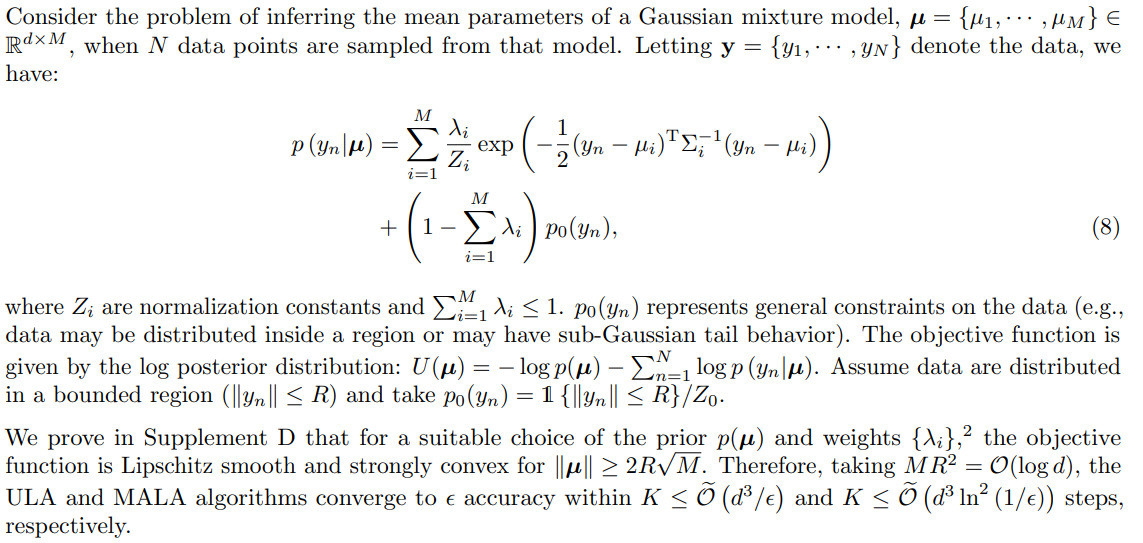### Questions about formulation of GMMs in paper

Hey all!

We are working on the project for the course and have decided to empirically validate some of the results of this paper. We will be comparing EM and ULA on a set of finite mixture modeling problems to see the number of gradient queries required with increasing $$d$$. The first part of the project is for us to simply replicate the results of that paper in the context of the Gaussian mixture modeling problem. The way it is presented in the paper is quite different from how it was presented in the class of machine learning last semester, so we have a couple of questions about that which we hope you could clear up for us. The formulation is as follows (on page 7 eq. 8):To us it seems as though this is a more general formulation, after which they place enough restrictions on the choice of parameters such that it reduces to the formulation we have seen in class (except with isotropic covariance matrices, and clusters placed such that they are unlikely to overlap). E.g.: the use of a constant mixture term makes sense from a probabilistic perspective, but it doesn't seem like it would affect the choice of optimal parameters. We would just like to see if this intuition is correct or if we are totally misinterpreting the problem statement in the paper?

$$\lambda_i$$ seems to generally correspond to the mixing parameters seen in the class of ML, is this true? And is it also true that they generally assigned the value of these to be $$\lambda_i = \sigma^2 Z_i / 1000$$ in the experiment? On a similar note: the normalization constant $$Z_i$$ also seems to be constant given the isotropic covariance matrices?

We are also confused about the m-parameter of the GMM formulation in the appendix: does it correspond to the $$m$$-strength convexity parameter seen in objective U in lemma 19 (p.35)? The lemma there assumed a radius $$R = 1/2$$, but the appendix specifies a radius of $$2\log_2 d$$, does that mean we also have to adjust m in our experiment?

Hopefully we have presented enough context for you to give us feedback, thanks in advance!

Top comment

Hi,

I am not familiar with the paper you shared, and haven't read it in detail.
What I can say about the $$\lambda_i$$, is that they correspond to $$\pi_i$$ in the ml course lecture notes. These values are usually learned from the data (I don't think setting it to $$\sigma^2 Z_i/1000$$ makes sense, but haven't looked at the experiment).

On a similar note: the normalization constant also seems to be constant given the isotropic covariance matrices?

Yes, it should be constant in that case.

Hope this is a little useful. Good luck!

Page 1 of 1